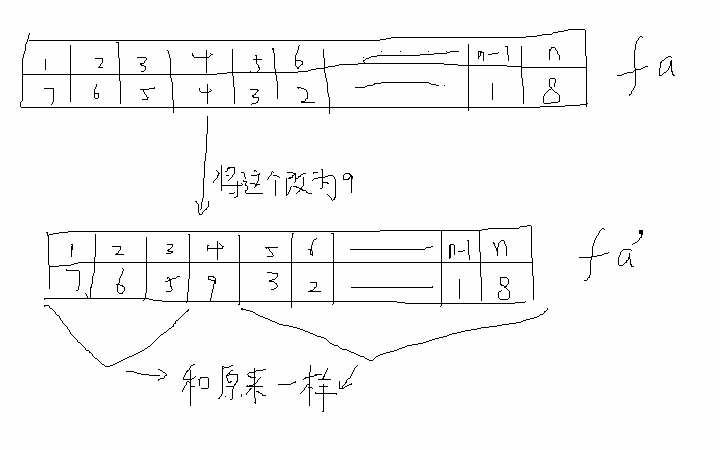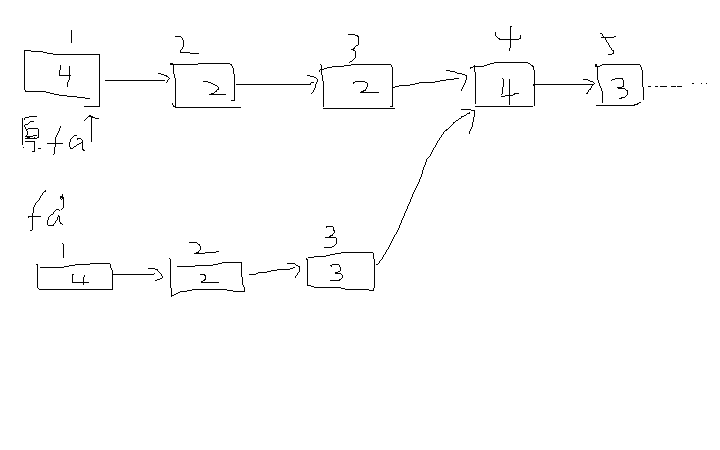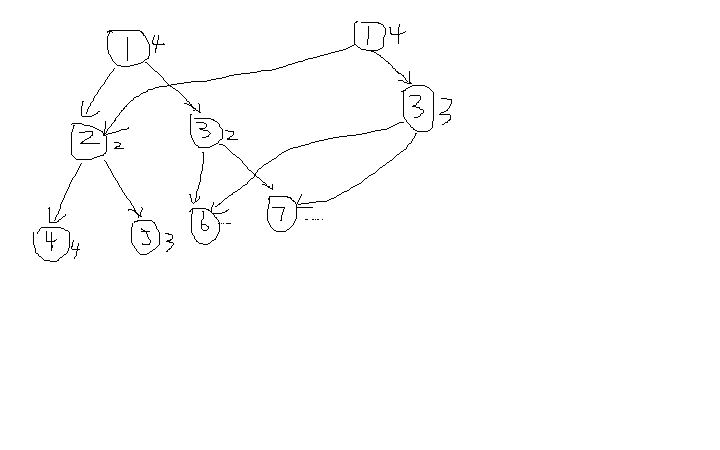# 关于可持久化并查集的学习和思考

## BZOJ3674：可持久化并查集加强版

Description

hzwer:乱写能AC，暴力踩标程
KuribohG：我不路径压缩就过了！
ndsf：暴力就可以轻松虐！
zky:……

n个集合 m个操作

1 a b 合并a,b所在集合
2 k 回到第k次操作之后的状态(查询算作操作)
3 a b 询问a,b是否属于同一集合，是则输出1否则输出0

0<n,m<=2*10^5#include<iostream>
#include<string>
#include<cstring>
#include<cmath>
#include<cstdio>
#include<cstdlib>
#include<stdio.h>
#include<algorithm>
using namespace std;

const int maxn=200100;
const int maxl=20;

struct data
{
int lson,rson,id,val,_Size;
} tree[maxn+2*maxn*maxl];
int Root[maxn];
int cur;

int w[maxn];
int n,m,lt,nt,lans;

void Build(int x)
{
tree[x].id=x;
tree[x].val=x;
tree[x]._Size=1;

int left=x<<1;
if (left<=n)
{
tree[x].lson=left;
Build(left);
}

int right=left|1;
if (right<=n)
{
tree[x].rson=right;
Build(right);
}
}

data Query(int root,int L,int x)
{
if (x==L) return tree[root];
if (x&(1<<(w[x]-w[L]-1))) return Query(tree[root].rson,L*2+1,x);
else return Query(tree[root].lson,L*2,x);
}

data Find_fa(int x)
{
data y=Query(Root[lt],1,x);
while (y.id!=y.val) y=Query(Root[lt],1,y.val);
return y;
}

void Update(int root,int L,int x,int nid,int v)
{
if (L==x)
{
if (nid==0) tree[root].val=v;
else tree[root]._Size=v;
return;
}
if (x&(1<<(w[x]-w[L]-1)))
{
data temp=tree[ tree[root].rson ];
tree[root].rson=++cur;
tree[cur]=temp;
Update(cur,L*2+1,x,nid,v);
}
else
{
data temp=tree[ tree[root].lson ];
tree[root].lson=++cur;
tree[cur]=temp;
Update(cur,L*2,x,nid,v);
}
}

{
data fx=Find_fa(x);
data fy=Find_fa(y);

if (fx.val==fy.val)
{
Root[nt]=Root[lt];
return;
}

if (fx._Size>fy._Size) swap(fx,fy);

Root[nt]=++cur;
tree[cur]=tree[ Root[lt] ];
Update(cur,1,fx.id,0,fy.id);

tree[++cur]=tree[ Root[nt] ];
Root[nt]=cur;
Update(cur,1,fy.id,1,fx._Size+fy._Size);
}

int main()
{
freopen("c.in","r",stdin);
freopen("c.out","w",stdout);

scanf("%d%d",&n,&m);

Build(1);

Root=1;
cur=n;
lt=1,nt=1;
lans=0;

w=1;
for (int i=2; i<maxn; i++) w[i]=w[i/2]+1;

/*for (int i=1; i<=n; i++)
{
data temp=Find_fa(i);
printf("%d ",temp.val);
}
printf("\n");*/

for (int i=1; i<=m; i++)
{
int op;
scanf("%d",&op);

if (op==1)
{
int a,b;
scanf("%d%d",&a,&b);
a=a^lans;
b=b^lans;

nt++;
lt=nt;
}

if (op==2)
{
int k;
scanf("%d",&k);
k=k^lans;

lt=k+1;
nt++;
Root[nt]=Root[lt];
lt=nt;
}

if (op==3)
{
int a,b;
scanf("%d%d",&a,&b);
a=a^lans;
b=b^lans;

//printf("%d %d\n",a,b);

data fa=Find_fa(a);
data fb=Find_fa(b);
bool f=(fa.val==fb.val);

printf("%d\n",f);
lans=f;

nt++;
Root[nt]=Root[lt];
lt=nt;
}

/*for (int i=1; i<=n; i++)
{
data temp=Find_fa(i);
printf("%d ",temp.val);
}
printf("\n");*/
}

return 0;
}©️2019 CSDN 皮肤主题: 技术黑板 设计师: CSDN官方博客